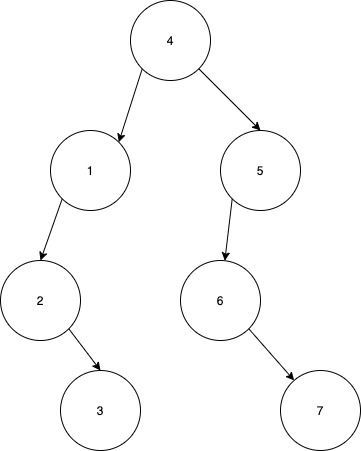New update is available. Click here to update.

# Maximum Difference Between Node And Ancestor

Last Updated: 13 Dec, 2020
Difficulty: Easy

## PROBLEM STATEMENT

#### For example :#### Some of them are, and their difference is:

``````|1-4|=3
|2-4|=2
|3-4|=1
|6-4|=2
|7-4|=3
And more
``````

#### Out of all the possible parent ancestor pairs, the one with the maximum absolute difference is between nodes (7 and 4) and (1 and 4) which is 3. Therefore, the answer to this case is 3.

##### Input Format :
``````The first line contains an Integer 'T' which denotes the number of test cases or queries to be run. Then the test cases follow.

The first line of input contains the elements of the tree in the level order form separated by a single space.

If any node does not have left or right child, take -1 in its place. Refer to the example below.

Example :

Elements are in the level order form. The input consists of values of nodes separated by a single space in a single line. In case a node is null, we take -1 on its place.

For example, the input for the tree depicted in the below image would be :
````````````1
2 3
4 -1 5 6
-1 7 -1 -1 -1 -1
-1 -1

Explanation :
Level 1 :
The root node of the tree is 1

Level 2 :
Left child of 1 = 2
Right child of 1 = 3

Level 3 :
Left child of 2 = 4
Right child of 2 = null (-1)
Left child of 3 = 5
Right child of 3 = 6

Level 4 :
Left child of 4 = null (-1)
Right child of 4 = 7
Left child of 5 = null (-1)
Right child of 5 = null (-1)
Left child of 6 = null (-1)
Right child of 6 = null (-1)

Level 5 :
Left child of 7 = null (-1)
Right child of 7 = null (-1)

The first not-null node (of the previous level) is treated as the parent of the first two nodes of the current level. The second not-null node (of the previous level) is treated as the parent node for the next two nodes of the current level and so on.

The input ends when all nodes at the last level are null (-1).
``````
##### Note :
``````The above format was just to provide clarity on how the input is formed for a given tree.

The sequence will be put together in a single line separated by a single space. Hence, for the above-depicted tree, the input will be given as:

1 2 3 4 -1 5 6 -1 7 -1 -1 -1 -1 -1 -1
``````
##### Output Format :
``````For each test case, return a single integer denoting the maximum difference between node and ancestor
``````
##### Note :
``````Do not print anything, just return the maximum difference between the node and the ancestor
``````
##### Constraints :
``````1 <= T <= 50
1<= N <= 10^3

Where 'N' denotes the number of nodes in the binary tree.

Time limit: 1 second
``````## Approach 1

• The idea is to traverse the binary tree and for each node check all its children and find the difference of the node with each of its children.
• This can be done by recursively checking for each node the maximum and minimum node in its left and right subtree and return its maximum difference over all nodes in the tree.

Keeping in mind the above idea, we can devise the following recursive  approach:

• Define 2 functions ‘maxInSub’ and ‘minInSub’ to calculate the maximum and minimum in any subtree for every node.
• We can calculate both the functions recursively by comparing the max or min with the current node and recursively calculating answer for the left and right subtree.
• Then, recursively check for each node for the maximum in its subtree and the minimum in its subtree and find the maximum difference.
• Finally, return the overall maximum difference value over all nodes of the tree.